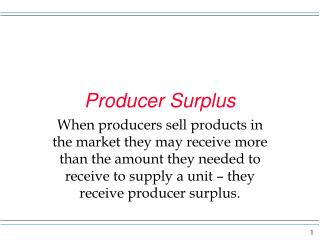DownloadDownload PresentationProducer Surplus

# Producer Surplus

Télécharger la présentation## Producer Surplus

- - - - - - - - - - - - - - - - - - - - - - - - - - - E N D - - - - - - - - - - - - - - - - - - - - - - - - - - -
##### Presentation Transcript

1. Producer Surplus When producers sell products in the market they may receive more than the amount they needed to receive to supply a unit – they receive producer surplus.

2. producer surplus Recall that the supply curve shows various prices and associated quantities producers would make available for sale. The amount sellers need to receive to induce them to make available for sale units of a product are located on the supply curve. The amount actually received is market determined. NOTE: as we try to learn about this concept is it useful to pick a quantity and look up vertically to the supply curve and see the price.

3. need to receive Notice at Q = 1 P1 is the lowest price at which the firm would supply Q = 1. P1 is not enough of an amount to have the 2nd unit supplied. To get 2 units supplied, P2 is required. P S P2 P1 a b c d e Q 1 2

4. need to receive On the previous slide we see that to get 1 unit supplied P1 was need. P1 times 1 = P1 and is the area of the rectangle made up of C and D. This is the amount needed to supply the first unit. If we ignore area c we could say the area under the supply up to 1 unit is the amount needed to get that unit supplied. You see the area under the curve is an under-estimate of the amount needed but it makes life easy in terms of a visual look. Similarly area b + e = P2, the amount needed to have the second unit supplied. If you ignore b we have just the area under the curve. The area under the supply curve out to a quantity is the amount needed to supply those units in the market.

5. P Here I picked “This Q” at random and I show you that triangle B represents the minimum payment needed to get the sellers to supply the “This Q.” S B Q This Q

6. P If the market price is “This P, “This Q” would be supplied by firms. The actual amount received by firms would be rectangle A + B and we would call this the total revenue of the firms involved. It is also called industry sales. S This P A B Q This Q

7. P Producer surplus here is area A. A is that part of revenue that is over and above the minimum payments (area B) needed to supply “This Q.” S This P A B Q This Q

8. Producer surplus P 25 10 With S & D we get P = 10 and Q = 300 Area A = .5(300)(10 - 4) =900 Area A + B = 10(300) = 3000 Area B = A + B – A = 2100 A B 4 300 Q Producers actually receive A + B = 3000, but only needed B = 2100, so the producer surplus is A = 900.

9. producer surplus What do producers do with the surplus received? They may use it to pay off some expenses or it could be a part of profit. Note: producer surplus and profit are not the same thing.